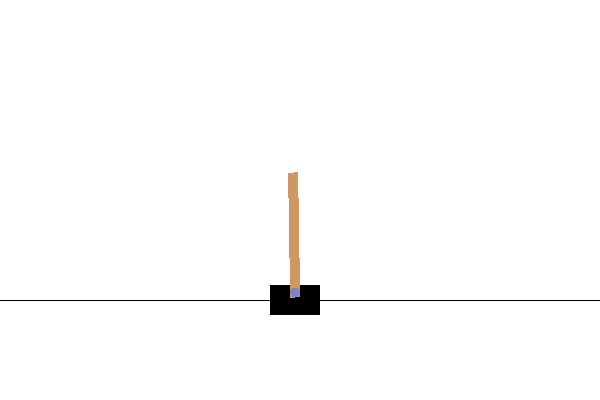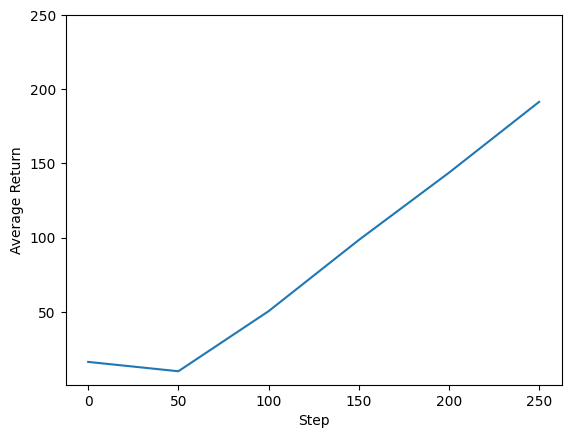# REINFORCE agent

## Introduction

This example shows how to train a REINFORCE agent on the Cartpole environment using the TF-Agents library, similar to the DQN tutorial.We will walk you through all the components in a Reinforcement Learning (RL) pipeline for training, evaluation and data collection.

## Setup

If you haven't installed the following dependencies, run:

````sudo apt-get update`
`sudo apt-get install -y xvfb ffmpeg freeglut3-dev`
`pip install 'imageio==2.4.0'`
`pip install pyvirtualdisplay`
`pip install tf-agents[reverb]`
`pip install pyglet xvfbwrapper`
```
``````from __future__ import absolute_import
from __future__ import division
from __future__ import print_function

import base64
import imageio
import IPython
import matplotlib.pyplot as plt
import numpy as np
import PIL.Image
import pyvirtualdisplay
import reverb

import tensorflow as tf

from tf_agents.agents.reinforce import reinforce_agent
from tf_agents.drivers import py_driver
from tf_agents.environments import suite_gym
from tf_agents.environments import tf_py_environment
from tf_agents.networks import actor_distribution_network
from tf_agents.policies import py_tf_eager_policy
from tf_agents.replay_buffers import reverb_replay_buffer
from tf_agents.replay_buffers import reverb_utils
from tf_agents.specs import tensor_spec
from tf_agents.trajectories import trajectory
from tf_agents.utils import common

# Set up a virtual display for rendering OpenAI gym environments.
display = pyvirtualdisplay.Display(visible=0, size=(1400, 900)).start()
``````

## Hyperparameters

``````env_name = "CartPole-v0" # @param {type:"string"}
num_iterations = 250 # @param {type:"integer"}
collect_episodes_per_iteration = 2 # @param {type:"integer"}
replay_buffer_capacity = 2000 # @param {type:"integer"}

fc_layer_params = (100,)

learning_rate = 1e-3 # @param {type:"number"}
log_interval = 25 # @param {type:"integer"}
num_eval_episodes = 10 # @param {type:"integer"}
eval_interval = 50 # @param {type:"integer"}
``````

## Environment

Environments in RL represent the task or problem that we are trying to solve. Standard environments can be easily created in TF-Agents using `suites`. We have different `suites` for loading environments from sources such as the OpenAI Gym, Atari, DM Control, etc., given a string environment name.

Now let us load the CartPole environment from the OpenAI Gym suite.

``````env = suite_gym.load(env_name)
``````

We can render this environment to see how it looks. A free-swinging pole is attached to a cart. The goal is to move the cart right or left in order to keep the pole pointing up.

``````env.reset()
PIL.Image.fromarray(env.render())
``````The `time_step = environment.step(action)` statement takes `action` in the environment. The `TimeStep` tuple returned contains the environment's next observation and reward for that action. The `time_step_spec()` and `action_spec()` methods in the environment return the specifications (types, shapes, bounds) of the `time_step` and `action` respectively.

``````print('Observation Spec:')
print(env.time_step_spec().observation)
print('Action Spec:')
print(env.action_spec())
``````
```Observation Spec:
BoundedArraySpec(shape=(4,), dtype=dtype('float32'), name='observation', minimum=[-4.8000002e+00 -3.4028235e+38 -4.1887903e-01 -3.4028235e+38], maximum=[4.8000002e+00 3.4028235e+38 4.1887903e-01 3.4028235e+38])
Action Spec:
BoundedArraySpec(shape=(), dtype=dtype('int64'), name='action', minimum=0, maximum=1)
```

So, we see that observation is an array of 4 floats: the position and velocity of the cart, and the angular position and velocity of the pole. Since only two actions are possible (move left or move right), the `action_spec` is a scalar where 0 means "move left" and 1 means "move right."

``````time_step = env.reset()
print('Time step:')
print(time_step)

action = np.array(1, dtype=np.int32)

next_time_step = env.step(action)
print('Next time step:')
print(next_time_step)
``````
```Time step:
TimeStep(
{'discount': array(1., dtype=float32),
'observation': array([-0.02294981,  0.03753006, -0.04879921, -0.02516104], dtype=float32),
'reward': array(0., dtype=float32),
'step_type': array(0, dtype=int32)})
Next time step:
TimeStep(
{'discount': array(1., dtype=float32),
'observation': array([-0.02219921,  0.23331663, -0.04930243, -0.33283257], dtype=float32),
'reward': array(1., dtype=float32),
'step_type': array(1, dtype=int32)})
```

Usually we create two environments: one for training and one for evaluation. Most environments are written in pure python, but they can be easily converted to TensorFlow using the `TFPyEnvironment` wrapper. The original environment's API uses numpy arrays, the `TFPyEnvironment` converts these to/from `Tensors` for you to more easily interact with TensorFlow policies and agents.

``````train_py_env = suite_gym.load(env_name)

train_env = tf_py_environment.TFPyEnvironment(train_py_env)
eval_env = tf_py_environment.TFPyEnvironment(eval_py_env)
``````

## Agent

The algorithm that we use to solve an RL problem is represented as an `Agent`. In addition to the REINFORCE agent, TF-Agents provides standard implementations of a variety of `Agents` such as DQN, DDPG, TD3, PPO and SAC.

To create a REINFORCE Agent, we first need an `Actor Network` that can learn to predict the action given an observation from the environment.

We can easily create an `Actor Network` using the specs of the observations and actions. We can specify the layers in the network which, in this example, is the `fc_layer_params` argument set to a tuple of `ints` representing the sizes of each hidden layer (see the Hyperparameters section above).

``````actor_net = actor_distribution_network.ActorDistributionNetwork(
train_env.observation_spec(),
train_env.action_spec(),
fc_layer_params=fc_layer_params)
``````

We also need an `optimizer` to train the network we just created, and a `train_step_counter` variable to keep track of how many times the network was updated.

``````optimizer = tf.keras.optimizers.Adam(learning_rate=learning_rate)

train_step_counter = tf.Variable(0)

tf_agent = reinforce_agent.ReinforceAgent(
train_env.time_step_spec(),
train_env.action_spec(),
actor_network=actor_net,
optimizer=optimizer,
normalize_returns=True,
train_step_counter=train_step_counter)
tf_agent.initialize()
``````
```2023-09-26 12:00:44.633379: W tensorflow/core/common_runtime/gpu/gpu_device.cc:1960] Cannot dlopen some GPU libraries. Please make sure the missing libraries mentioned above are installed properly if you would like to use GPU. Follow the guide at https://www.tensorflow.org/install/gpu for how to download and setup the required libraries for your platform.
Skipping registering GPU devices...
```

## Policies

In TF-Agents, policies represent the standard notion of policies in RL: given a `time_step` produce an action or a distribution over actions. The main method is `policy_step = policy.action(time_step)` where `policy_step` is a named tuple `PolicyStep(action, state, info)`. The `policy_step.action` is the `action` to be applied to the environment, `state` represents the state for stateful (RNN) policies and `info` may contain auxiliary information such as log probabilities of the actions.

Agents contain two policies: the main policy that is used for evaluation/deployment (agent.policy) and another policy that is used for data collection (agent.collect_policy).

``````eval_policy = tf_agent.policy
collect_policy = tf_agent.collect_policy
``````

## Metrics and Evaluation

The most common metric used to evaluate a policy is the average return. The return is the sum of rewards obtained while running a policy in an environment for an episode, and we usually average this over a few episodes. We can compute the average return metric as follows.

``````def compute_avg_return(environment, policy, num_episodes=10):

total_return = 0.0
for _ in range(num_episodes):

time_step = environment.reset()
episode_return = 0.0

while not time_step.is_last():
action_step = policy.action(time_step)
time_step = environment.step(action_step.action)
episode_return += time_step.reward
total_return += episode_return

avg_return = total_return / num_episodes
return avg_return.numpy()

# Please also see the metrics module for standard implementations of different
# metrics.
``````

## Replay Buffer

In order to keep track of the data collected from the environment, we will use Reverb, an efficient, extensible, and easy-to-use replay system by Deepmind. It stores experience data when we collect trajectories and is consumed during training.

This replay buffer is constructed using specs describing the tensors that are to be stored, which can be obtained from the agent using `tf_agent.collect_data_spec`.

``````table_name = 'uniform_table'
replay_buffer_signature = tensor_spec.from_spec(
tf_agent.collect_data_spec)
replay_buffer_signature)
table = reverb.Table(
table_name,
max_size=replay_buffer_capacity,
sampler=reverb.selectors.Uniform(),
remover=reverb.selectors.Fifo(),
rate_limiter=reverb.rate_limiters.MinSize(1),
signature=replay_buffer_signature)

reverb_server = reverb.Server([table])

replay_buffer = reverb_replay_buffer.ReverbReplayBuffer(
tf_agent.collect_data_spec,
table_name=table_name,
sequence_length=None,
local_server=reverb_server)

replay_buffer.py_client,
table_name,
replay_buffer_capacity
)
``````
```[reverb/cc/platform/tfrecord_checkpointer.cc:162]  Initializing TFRecordCheckpointer in /tmpfs/tmp/tmp_vvwecse.
[reverb/cc/platform/default/server.cc:71] Started replay server on port 42653
```

For most agents, the `collect_data_spec` is a `Trajectory` named tuple containing the observation, action, reward etc.

## Data Collection

As REINFORCE learns from whole episodes, we define a function to collect an episode using the given data collection policy and save the data (observations, actions, rewards etc.) as trajectories in the replay buffer. Here we are using 'PyDriver' to run the experience collecting loop. You can learn more about TF Agents driver in our drivers tutorial.

``````def collect_episode(environment, policy, num_episodes):

driver = py_driver.PyDriver(
environment,
py_tf_eager_policy.PyTFEagerPolicy(
policy, use_tf_function=True),
[rb_observer],
max_episodes=num_episodes)
initial_time_step = environment.reset()
driver.run(initial_time_step)
``````

## Training the agent

The training loop involves both collecting data from the environment and optimizing the agent's networks. Along the way, we will occasionally evaluate the agent's policy to see how we are doing.

The following will take ~3 minutes to run.

``````try:
%%time
except:
pass

# (Optional) Optimize by wrapping some of the code in a graph using TF function.
tf_agent.train = common.function(tf_agent.train)

# Reset the train step
tf_agent.train_step_counter.assign(0)

# Evaluate the agent's policy once before training.
avg_return = compute_avg_return(eval_env, tf_agent.policy, num_eval_episodes)
returns = [avg_return]

for _ in range(num_iterations):

# Collect a few episodes using collect_policy and save to the replay buffer.
collect_episode(
train_py_env, tf_agent.collect_policy, collect_episodes_per_iteration)

# Use data from the buffer and update the agent's network.
iterator = iter(replay_buffer.as_dataset(sample_batch_size=1))
trajectories, _ = next(iterator)
train_loss = tf_agent.train(experience=trajectories)

replay_buffer.clear()

step = tf_agent.train_step_counter.numpy()

if step % log_interval == 0:
print('step = {0}: loss = {1}'.format(step, train_loss.loss))

if step % eval_interval == 0:
avg_return = compute_avg_return(eval_env, tf_agent.policy, num_eval_episodes)
print('step = {0}: Average Return = {1}'.format(step, avg_return))
returns.append(avg_return)
``````
```[reverb/cc/client.cc:165] Sampler and server are owned by the same process (24739) so Table uniform_table is accessed directly without gRPC.
[reverb/cc/client.cc:165] Sampler and server are owned by the same process (24739) so Table uniform_table is accessed directly without gRPC.
[reverb/cc/client.cc:165] Sampler and server are owned by the same process (24739) so Table uniform_table is accessed directly without gRPC.
[reverb/cc/client.cc:165] Sampler and server are owned by the same process (24739) so Table uniform_table is accessed directly without gRPC.
[reverb/cc/client.cc:165] Sampler and server are owned by the same process (24739) so Table uniform_table is accessed directly without gRPC.
step = 25: loss = 1.3191242218017578
[reverb/cc/client.cc:165] Sampler and server are owned by the same process (24739) so Table uniform_table is accessed directly without gRPC.
step = 50: loss = 1.252702236175537
step = 50: Average Return = 62.70000076293945
[reverb/cc/client.cc:165] Sampler and server are owned by the same process (24739) so Table uniform_table is accessed directly without gRPC.
step = 75: loss = 1.5663279294967651
step = 100: loss = 1.0793800354003906
step = 100: Average Return = 96.80000305175781
step = 125: loss = 1.089087724685669
[reverb/cc/client.cc:165] Sampler and server are owned by the same process (24739) so Table uniform_table is accessed directly without gRPC.
step = 150: loss = 0.6324303150177002
step = 150: Average Return = 111.69999694824219
step = 175: loss = 1.1537282466888428
step = 200: loss = 1.477043628692627
step = 200: Average Return = 106.30000305175781
step = 225: loss = 1.2247202396392822
step = 250: loss = 1.4869199991226196
step = 250: Average Return = 119.30000305175781
```

## Visualization

### Plots

We can plot return vs global steps to see the performance of our agent. In `Cartpole-v0`, the environment gives a reward of +1 for every time step the pole stays up, and since the maximum number of steps is 200, the maximum possible return is also 200.

``````steps = range(0, num_iterations + 1, eval_interval)
plt.plot(steps, returns)
plt.ylabel('Average Return')
plt.xlabel('Step')
plt.ylim(top=250)
``````
```(4.640000247955322, 250.0)
```### Videos

It is helpful to visualize the performance of an agent by rendering the environment at each step. Before we do that, let us first create a function to embed videos in this colab.

``````def embed_mp4(filename):
"""Embeds an mp4 file in the notebook."""
b64 = base64.b64encode(video)
tag = '''
<video width="640" height="480" controls>
<source src="data:video/mp4;base64,{0}" type="video/mp4">
Your browser does not support the video tag.
</video>'''.format(b64.decode())

return IPython.display.HTML(tag)
``````

The following code visualizes the agent's policy for a few episodes:

``````num_episodes = 3
video_filename = 'imageio.mp4'
with imageio.get_writer(video_filename, fps=60) as video:
for _ in range(num_episodes):
time_step = eval_env.reset()
video.append_data(eval_py_env.render())
while not time_step.is_last():
action_step = tf_agent.policy.action(time_step)
time_step = eval_env.step(action_step.action)
video.append_data(eval_py_env.render())

embed_mp4(video_filename)
``````
```WARNING:root:IMAGEIO FFMPEG_WRITER WARNING: input image is not divisible by macro_block_size=16, resizing from (400, 600) to (400, 608) to ensure video compatibility with most codecs and players. To prevent resizing, make your input image divisible by the macro_block_size or set the macro_block_size to None (risking incompatibility). You may also see a FFMPEG warning concerning speedloss due to data not being aligned.
[swscaler @ 0x55a5fce73880] Warning: data is not aligned! This can lead to a speed loss
```
[{ "type": "thumb-down", "id": "missingTheInformationINeed", "label":"Missing the information I need" },{ "type": "thumb-down", "id": "tooComplicatedTooManySteps", "label":"Too complicated / too many steps" },{ "type": "thumb-down", "id": "outOfDate", "label":"Out of date" },{ "type": "thumb-down", "id": "samplesCodeIssue", "label":"Samples / code issue" },{ "type": "thumb-down", "id": "otherDown", "label":"Other" }]
[{ "type": "thumb-up", "id": "easyToUnderstand", "label":"Easy to understand" },{ "type": "thumb-up", "id": "solvedMyProblem", "label":"Solved my problem" },{ "type": "thumb-up", "id": "otherUp", "label":"Other" }]